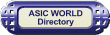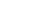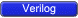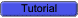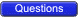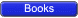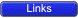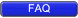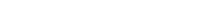Combinational UDPs In combinational UDPs, the output is determined as a function of the current input. Whenever an input changes value, the UDP is evaluated and one of the state table rows is matched. The output state is set to the value indicated by that row. This is similar to condition statements: each line in table is one condition.Combinational UDPs have one field per input and one field for the output. Input fields and output fields are separated with colon. Each row of the table is terminated by a semicolon. For example, the following state table entry specifies that when the three inputs are all 0, the output is 0.``` 1 primitive udp_combo (.....); 2 3 table 4 0 0 0 : 0; 5 ... 6 endtable 7 8 endprimitive ``` You could download file udp_combo.v hereThe order of the inputs in the state table description must correspond to the order of the inputs in the port list in the UDP definition header. It is not related to the order of the input declarations.Each row in the table defines the output for a particular combination of input states. If all inputs are specified as x, then the output must be specified as x. All combinations that are not explicitly specified result in a default output state of x.Example In the below example entry, the ? represents a don't-care condition. This symbol indicates iterative substitution of 1, 0, and x. The table entry specifies that when the inputs are 0 and 1, the output is 1 no matter what the value of the current state is.You do not have to explicitly specify every possible input combination. All combinations that are not explicitly specified result in a default output state of x.It is illegal to have the same combination of inputs, specified for different outputs.``` 1 // This code shows how UDP body looks like 2 primitive udp_body ( 3 a, // Port a 4 b, // Port b 5 c // Port c 6 ); 7 output a; 8 input b,c; 9 10 // UDP function code here 11 // A = B | C; 12 table 13 // B C : A 14 ? 1 : 1; 15 1 ? : 1; 16 0 0 : 0; 17 endtable 18 19 endprimitive ``` You could download file udp_body.v hereTestBench to check above UDP``` 1 `include "udp_body.v" 2 module udp_body_tb(); 3 4 reg b,c; 5 wire a; 6 7 udp_body udp (a,b,c); 8 9 initial begin 10 \$monitor(" B = %b C = %b A = %b",b,c,a); 11 b = 0; 12 c = 0; 13 #1 b = 1; 14 #1 b = 0; 15 #1 c = 1; 16 #1 b = 1'bx; 17 #1 c = 0; 18 #1 b = 1; 19 #1 c = 1'bx; 20 #1 b = 0; 21 #1 \$finish; 22 end 23 24 endmodule ``` You could download file udp_body_tb.v hereSimulator Output``` B = 0 C = 0 A = 0 B = 1 C = 0 A = 1 B = 0 C = 0 A = 0 B = 0 C = 1 A = 1 B = x C = 1 A = 1 B = x C = 0 A = x B = 1 C = 0 A = 1 B = 1 C = x A = 1 B = 0 C = x A = x ```Copyright © 1998-2014 Deepak Kumar Tala - All rights reserved Do you have any Comment? mail me at:deepak@asic-world.com